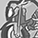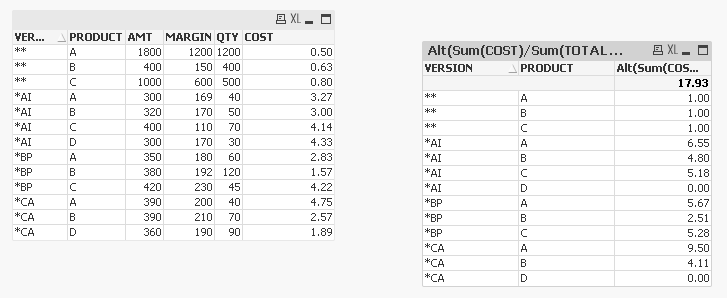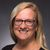# New to Qlik Sense

If you’re new to Qlik Sense, start with this Discussion Board and get up-to-speed quickly.

Announcements
Support Case Portal has moved to Qlik Community! Read the FAQs to start exploring Support resources.
cancel
Showing results for
Did you mean:Contributor III

## DIVISION BY FIXED value of a variable

Hello,

I am trying to divide COST column by COST of VERSION=**. i am using below code for that.

[ (Sum({<VG={"POS"},METRIC={"AMT \$"}>}VALUE)/1000000)   - (Sum({<VG={"POS"},METRIC={"MARGIN \$"}>}VALUE)/1000000) )

/

(Sum({<VG={"POS"},METRIC={"QTY #"}>}VALUE)/10000 ]

/

[  (Sum({<VG={"POS"},VERSION={"**"},METRIC={"AMT \$"}>}VALUE)/1000000)    -  (Sum({<VG={"POS"},VERSION={"**"},METRIC={"MARGIN \$"}>}VALUE)/1000000) )

/

(Sum({<VG={"POS"},VERSION={"**"},METRIC={"QTY #"}>}VALUE)/10000 ]

But, the above code is doing COST/COST - for respective VERSIONS. But I need all the costs should be divided by hte cost of VERSION = ** .

 VERSION AMT MARGIN QTY COST (AMT-MARGIN/QTY) GETTING BUT I NEED ** \$3,195.15 \$1,635.47 2,659 \$0.59 \$1.00 \$1.00 *AI \$544.54 \$377.88 149 \$1.12 \$1.00 \$1.91 *BP \$584.71 \$355.52 345 \$0.66 \$1.00 \$1.13 *CA \$1,441.26 \$764.90 612 \$1.10 \$1.00 \$1.88

How can i achieve the result of

COST/Cost of VERSION=**.

Thanks.

Labels (2)

• ### FIXED DIVISION

2 Solutions

Accepted SolutionsMVP

Try this

```((Sum({<VG = {"POS"}, METRIC = {"AMT \$"}>} VALUE)/1000000) - (Sum({<VG = {"POS"}, METRIC = {"MARGIN \$"}>} VALUE)/1000000))
/
(Sum({<VG = {"POS"}, METRIC = {"QTY #"}>} VALUE)/10000)

/

((Sum(TOTAL {<VG = {"POS"}, VERSION = {'**'}, METRIC = {"AMT \$"}>} VALUE)/1000000) - (Sum(TOTAL {<VG = {"POS"}, VERSION = {'**'}, METRIC = {"MARGIN \$"}>} VALUE)/1000000))
/
(Sum(TOTAL {<VG = {"POS"}, VERSION = {'**'}, METRIC = {"QTY #"}>} VALUE)/10000)```MVP

Based on the sample provided... this type of expression should work

`Alt(Sum(COST)/Sum(TOTAL <PRODUCT> {<VERSION = {'**'}>} COST), 0)`4 RepliesMVP

Try this

```((Sum({<VG = {"POS"}, METRIC = {"AMT \$"}>} VALUE)/1000000) - (Sum({<VG = {"POS"}, METRIC = {"MARGIN \$"}>} VALUE)/1000000))
/
(Sum({<VG = {"POS"}, METRIC = {"QTY #"}>} VALUE)/10000)

/

((Sum(TOTAL {<VG = {"POS"}, VERSION = {'**'}, METRIC = {"AMT \$"}>} VALUE)/1000000) - (Sum(TOTAL {<VG = {"POS"}, VERSION = {'**'}, METRIC = {"MARGIN \$"}>} VALUE)/1000000))
/
(Sum(TOTAL {<VG = {"POS"}, VERSION = {'**'}, METRIC = {"QTY #"}>} VALUE)/10000)```Contributor III
Author

Thank you Sunny, That helped.

I am alos stuck with another thing in the similar prolem, there is a new column PRODUCT.

and i need to take care of it while divided by...

like, Cost of VERSION = AI , PRODUCT = A should be divided by COST of VERSION =**, PRODUCT = A and so on.

here is the table explanation.

 VERSION PRODUCT AMT MARGIN QTY COST (AMT-MARGIN/QTY) NEED HOW ? ** A 1800 1200 1200 \$                   0.50 1.00 COST( **,A )  /   COST(**,A) ** B 400 150 400 \$                   0.63 1.00 COST( **,B)   /   COST(**,B) ** C 1000 600 500 \$                   0.80 1.00 COST( **,C)   /   COST(**,C) *AI A 300 169 40 \$                   3.28 6.55 COST( *AI,A )  /   COST(**,A) *AI B 320 170 50 \$                   3.00 4.80 COST( *AI,B)   /   COST(**,B) *AI C 400 110 70 \$                   4.14 5.18 COST( *AI,C)   /   COST(**,C) *AI D 300 170 30 \$                   4.33 0.00 COST( *AI,D)   /   COST(**,D)[**, D not exists] *BP A 350 180 60 \$                   2.83 5.67 COST( *BP,A )  /   COST(**,A) *BP B 380 192 120 \$                   1.57 2.51 COST( *BP,B)   /   COST(**,B) *BP C 420 230 45 \$                   4.22 5.28 COST( *BP,C)   /   COST(**,C) *CA A 390 200 40 \$                   4.75 9.50 COST( *CA,A   /   COST(**,A) *CA B 390 210 70 \$                   2.57 4.11 COST( *CA,B)   /   COST(**,B) *CA D 360 190 90 \$                   1.89 0.00 COST( *CA,D)   /   COST(**,D)MVP

Based on the sample provided... this type of expression should work

`Alt(Sum(COST)/Sum(TOTAL <PRODUCT> {<VERSION = {'**'}>} COST), 0)`Community Manager
Is this QlikView? I would like to confirm and move this thread to the QlikView forum. Thanks
Sue MacalusoTags
Community Browser